The SURVEYLOGISTIC Procedure

### Notation

Let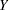be the response variable with categories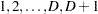. Thecovariates are denoted by a-dimension row vector.

For a stratified clustered sample design, each observation is represented by a row vector,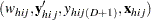, where

•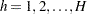is the stratum index

•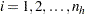is the cluster index within stratum•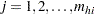is the unit index within clusterof stratum•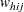denotes the sampling weight

•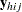is a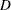-dimensional column vector whose elements are indicator variables for the firstcategories for variable. If the response of theth unit of theth cluster in stratumfalls in category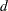, theth element of the vector is one, and the remaining elements of the vector are zero, where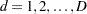.

•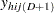is the indicator variable for the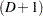category of variable•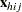denotes the-dimensional row vector of explanatory variables for theth unit of theth cluster in stratum. If there is an intercept, then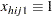.

•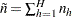is the total number of clusters in the sample

•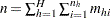is the total sample size

The following notations are also used:

•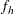denotes the sampling rate for stratum•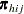is the expected vector of the response variable: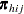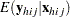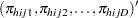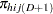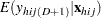Note that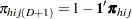, where 1 is a-dimensional column vector whose elements are.Previous Page | Next Page | Top of Page